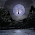## Friday, March 30, 2012

### Cymatics, Precession, Time and the Platonic Solids

Learning about Sacred Geometry has happened to me in a series of  mind-blowing epiphanies. I still don't understand it all and never will, but I am beginning to understand enough to see a pattern of creation.

Matter is energy and energy is vibration. According to John Stuart Reed, an Acoustical Engineer in England, Using cymatics the Frequency of 432 Hertz creates equilateral triangles, which are integral to the Flower of Life and the Platonic Solids.
Here are the different frequencies.
"When A is tuned to 432 Hz the frequencies of the other A’s shift to 27 Hz, 54, 108, 216, 864, 1728 in other octaves.
D becomes 576 Hz which becomes 9 Hz, 18, 36, 72, 144, 288, 1152 in other octaves.
E becomes 324 Hz which becomes 81 Hz, 162, 648, 1296 in other octaves. etc etc."

This is a good site to get the basics of cymatics.

When I saw the above numbers, I instantly recognized the numbers relative to time, precession and the platonic solids.

I am not good with music but below are hertz in basic notes in different octaves that create cymatic triangles.
27, 54, 108, 216,432, 864, 1728
9, 18, 36, 72, 144, 288, 576 1152
81, 162, 324, 648, 1296
Now let’s compare the above triangle creating cymatic frequencies with the platonic solids.

Tetrahedron has 4 sides with 180 degrees of angle per side making 720 Degrees total. Note the 18 and 72 above

Octahedron has 8 sides with 180 degrees of angle per side making 1440 Degrees total. Note the 18 and 144 above.

Cube has 6 sides with 360 degrees of angle per side making 2160 Degrees total. Note the 36 and 216 above.

Icosahedron  has 20 sides with 180 degrees of angle per side making 3600 Degrees total. Note the 18 and 36 above.

Dodecahedron  has 12 sides with 108 degrees per angle and 540 degrees of angle per side making  6480 Degrees total. Note the 108, 54 and 648 above

Are you beginning to see a relationship, between frequency and form?

Let’s compare.
Cymatic Frequency Numbers
27, 54108216,432864, 1728
9, 183672144, 288, 576 1152
81, 162, 324, 648, 1296

Degrees of angle in sides and total in Platonic Solids
108180, 2160, 360,540, 720, 1440, 3600, 6480

Now let’s look at time.  There are 3600 seconds in and hour,1440 minutes in a day 86400 seconds in a day, 2160 years in a zodiac age, 144000 days in a Mayan Baktun, 1080 parts to a Hebrew calendar hour. 1/2 of a Platonic Year is 12960 years, ¼ = 6480 years(the zodiac is divided into 4 parts), 1/3 = 8640 years.

Let’s consider Astronomy for a moment.  Diameter of Sun is 864000 miles, Radius is 432000. Diameter of Moon is2160 miles, radius is 1080. The Earth is orbiting the Sun at a speed of 108,000 Kilometers per hour or 2592000 in a 24 Hour Day. D of Sun 864000 * 108 = 93312000, the distance of the Sun from the Earth. 93312000/360 days(many ancient calendars) = 259200, the number of years in precession * 10. The Solar System is moving through the Milky Way Galaxy at 72000 KM per Hour or 1728000 per day. Precession moves by 1 degree every 72years. 72 x 360 degrees = 25920. A side note here is that in the Emerald Tablet of Thoth, the Moon is the Mother and the Sun is the Father. May be what it really means is that reality is constructed with frequencies related to the Sun and Moon measurements.
I could go on, but my point is show there is a numerical relationship between the precessional cycle, time, the platonic solids and Earth, Moon and Sun measurement.
You are looking at the program code

#### 1 comment:

1.Hi there

I just read through your article and found it a wonderful read. I'd also like to thank you for linking to my site.

Namaste

Timberwolf
www.timberwolfhq.com